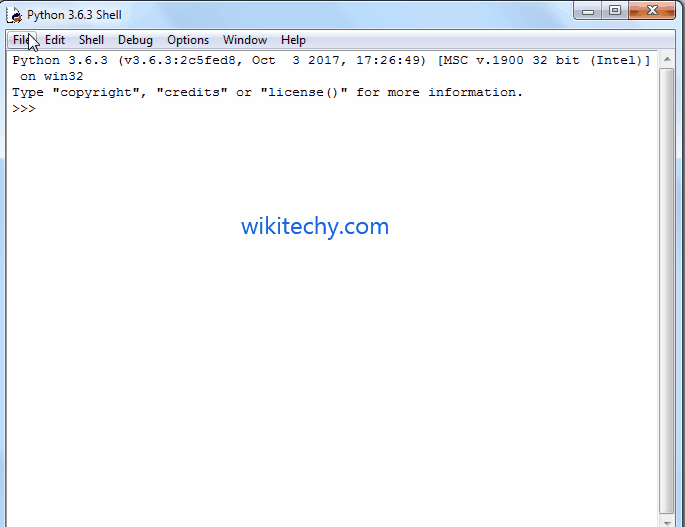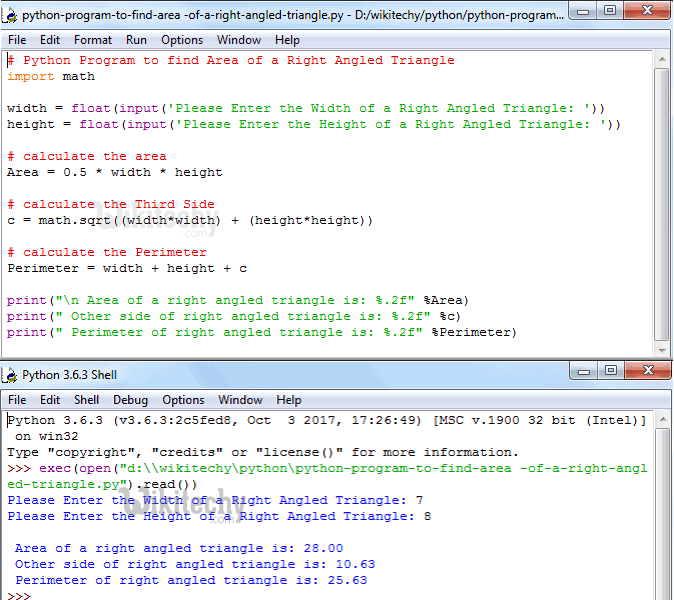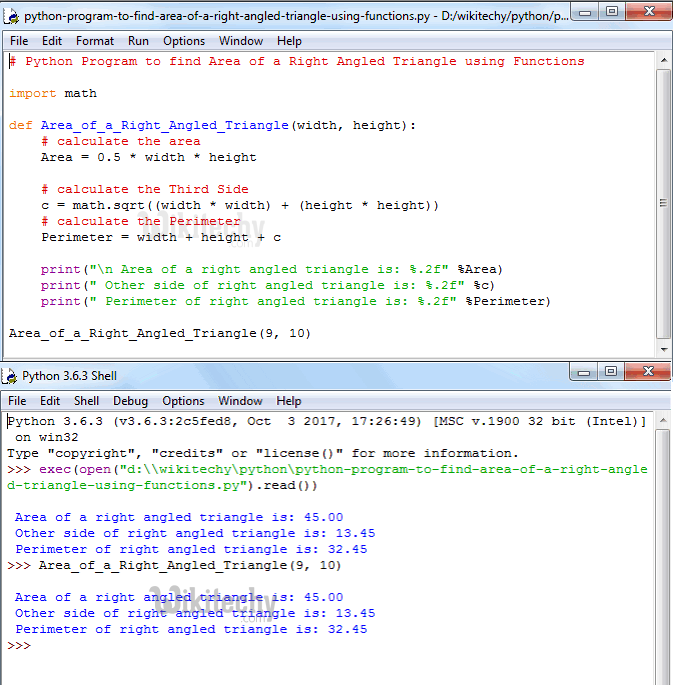# python tutorial - Python Program to find Area of a Right Angled Triangle - learn python - python programming

• To write Python Program to find Area of a Right Angled Triangle with example.
• Before we step into the program, Let see the definition and formula behind Area of a Right Angled TriangleLearn Python - Python tutorial - Python Program to find Area of a Right Angled Triangle - Python examples - Python programs

## Area of a Right Angled Triangle

• If we know the width and height then, we can calculate the area of a right angled triangle using below formula.
• Using Pythagoras formula we can easily find the unknown sides in the right angled triangle.
• Perimeter is the distance around the edges. We can calculate perimeter using below formula

## Python Program to find Area of a Right Angled Triangle

• This program allows the user to enter width and height of the right angled triangle.
• Using those values we will calculate the Area and perimeter of the right angled triangle.

## OutputLearn Python - Python tutorial - Python Program to find Area of a Right Angled Triangle - Python examples - Python programs

## Analysis

• First, We imported the math library using the following statement. This will allow us to use the mathematical functions like math.sqrt function
• Following statements will allow the User to enter the Width and Height of a right angled triangle.
• Next, we are calculating the area (The value of 1/2 = 0.5). So we used 0.5 * width*height as the formula
• In the next line, We are calculating the other side of a right-angled triangle using the Pythagoras formula C²=a²+b² , Which is similar to C = √a²+b²
• Here we used sqrt() function to calculate the square root of the a²+b². sqrt() is the math function, which is used to calculate the square root.
• In the next line, We are calculating the perimeter using the formula
• Following print statements will help us to print the Perimeter, Other side and Area of a right angled Triangle

## Python Program to find Area of a Right Angled Triangle using functions

• This program allows the user to enter the width and height of a right angled triangle.
• We will pass those values to the function arguments to calculate the area of a right angled triangle.

## OutputLearn Python - Python tutorial - Python Program to find Area of a Right Angled Triangle using functions - Python examples - Python programs

## Analysis

• First, We defined the function with two arguments using def keyword.
• It means, User will enter the width and height of a right angled triangle.
• Next, We are Calculating the Area of a right angled triangle as we described in our first example.

NOTE: We can call the function with arguments in .py file directly or else we can call it from the python shell. Please don’t forget the function arguments